Engineering ToolBox - Resources, Tools and Basic Information for Engineering and Design of Technical Applications!

# Geometric Shapes - Areas

## Areas, diagonals and more - of geometric figures like rectangles, triangles, trapezoids ..

### Square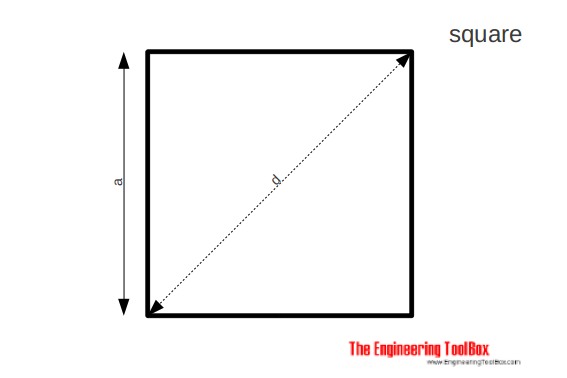The area of a square can be calculated as

A = a2                            (1a)

The side of a square can be calculated as

a = A1/2                            (1b)

The diagonal of a square can be calculated as

d = a 21/2                        (1c)

### Rectangle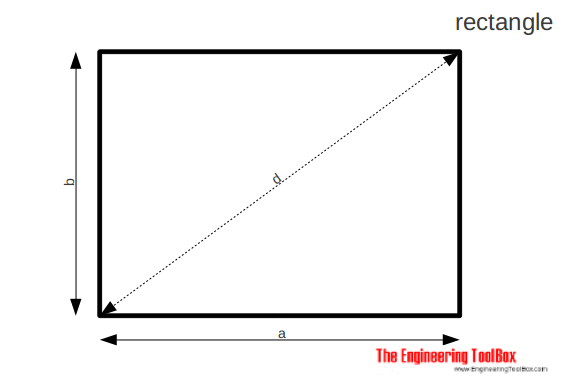The area of a rectangle can be calculated as

A = a b          (2a)

The diagonal of a rectangle can be calculated as

d = (a2 + b2)1/2     (2b)

### Parallelogram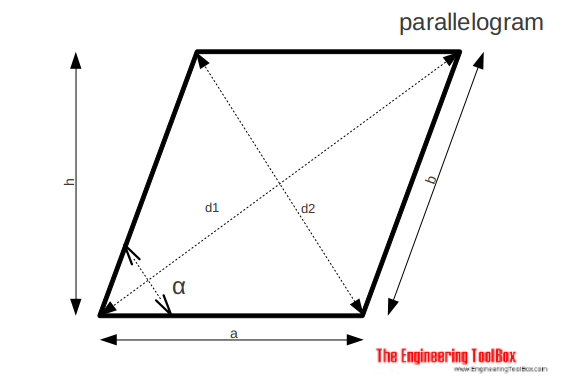The area of a parallelogram can be calculated as

A = a h

= a b sin α                        (3a)

The diameters of a parallelogram can be calculated as

d1 = ((a + h cot α)2 + h2)1/2                      (3b)

d2 = ((a - h cot α)2 + h2)1/2                     (3b)

### Equilateral Triangle

An equilateral triangle is a triangle in which all three sides are equal.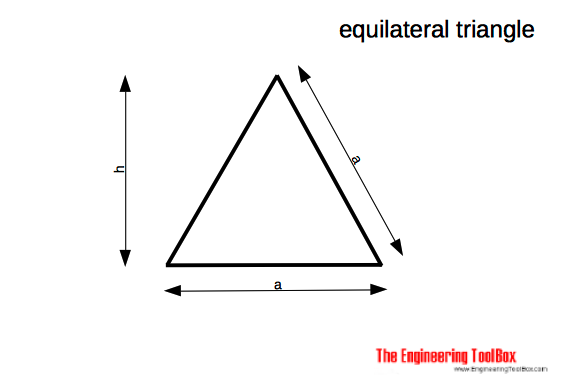The area of an equilateral triangle can be calculated as

A = a2/3 31/2                                 (4a)

The area of an equilateral triangle can be calculated as

h = a/2 31/2                              (4b)

### Triangle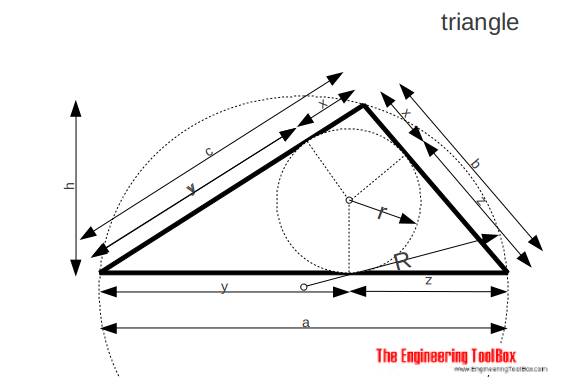The area of a triangle can be calculated as

A = a h / 2

= r s                                 (5a)

r = a h / 2s                          (5b)

R = b c / 2 h                        (5c)

s = (a + b + c) / 2                     (5d)

x = s - a                           (5e)

y = s - b                           (5f)

z = s - c                          (5g)

### Trapezoid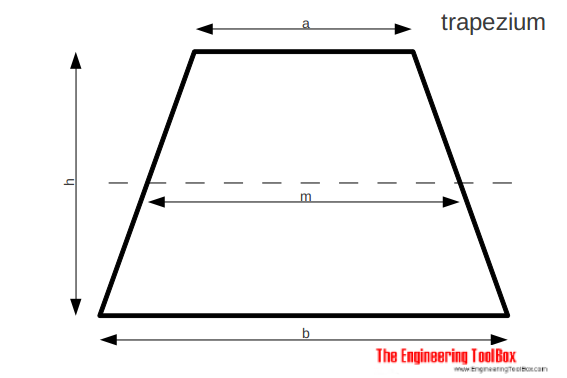The area of a trapezoid can be calculated as

A = 1/2 (a + b) h

= m h                           (6a)

m = (a + b) / 2                      (6b)

### Hexagon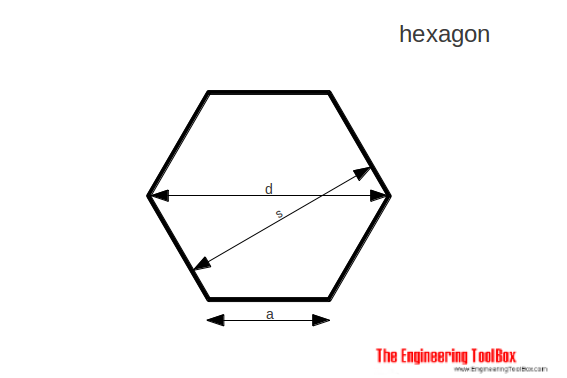The area of a hexagon can be calculated as

A = 3/2 a2 31/2                             (7a)

d = 2 a

=  2 / 31/2

= 1.1547005 s                              (7b)

s = 31/2 / 2 d

= 0.866025 d                              (7c)

### Circle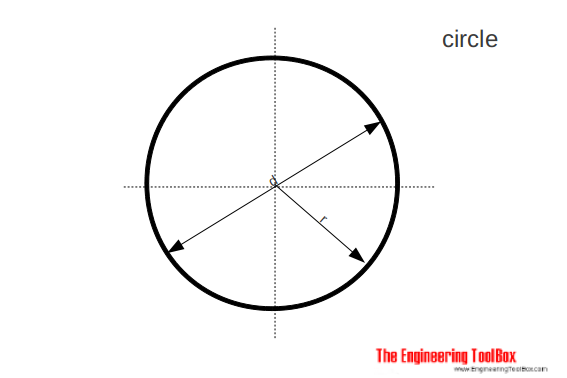The area of a circle can be calculated as

A = π/4 d2

= π r2

= 0.785.. d2                       (8a)

C = 2 π r

=  π d                           (8b)

where

C = circumference

### Sector and Segment of a Circle

#### Sector of Circle

Area of a sector of circle can be expressed as

A = 1/2 θr r2                            (9)

= 1/360 θd π r2

where

θd = angle in degrees

#### Segment of Circle

Area of a segment of circle can be expressed as

A = 1/2 (θr - sin θr) r2

= 1/2 (π θd/180 - sin θd) r2                             (10)

### Right Circular Cylinder

Lateral surface area of a right circular circle can be expressed as

A = 2 π r h                                      (11)

where

h = height of cylinder (m, ft)

r = radius of base (m, ft)

### Right Circular Cone

Lateral surface area of a right circular cone can be expressed as

A = π r l

= π r (r2 + h2)1/2                                   (12)

where

h = height of cone (m, ft)

r = radius of base (m, ft)

l = slant length (m, ft)

### Sphere

Lateral surface area of a sphere can be expressed as

A = 4 π r2                                    (13)

## Related Topics

• ### Mathematics

Mathematical rules and laws - numbers, areas, volumes, exponents, trigonometric functions and more.

## Related Documents

• ### Area of Intersecting Circles

Calculate area of intersecting circles
• ### Area Units Converter

Convert between units of area.
• ### Centroids of Plane Areas

The controid of square, rectangle, circle, semi-circle and right-angled triangle.
• ### Circle - Equation

The equation for a circle
• ### Circle - the Chord Lengths when Divided in to Equal Segments

Calculate chord lengths when dividing the circumference of a circle into an equal number of segments.
• ### Circles - Circumferences and Areas

Circumferences and areas of circles with diameters in inches.
• ### Circles Outside a Circle

Calculate the numbers of circles on the outside of an inner circle - like the geometry of rollers on a shaft.
• ### Cylindrical Tanks - Volumes

Volume in US gallons and liters.
• ### Elementary Curves

Ellipse, circle, hyperbola, parabola, parallel, intersecting and coincident lines.
• ### Equal Areas - Circles vs. Squares

Radius and side lengths of equal areas, circles and squares.
• ### Exponents - Powers and Roots

The laws of fractional and integer exponents.
• ### Factorials

The product of all positive integers.
• ### Hexagons and Squares - Diagonal Lengths

Distances between corners for hexagons and squares.
• ### Oblique Triangle

Calculate oblique triangles.
• ### Pythagorean Theorem

Verifying square corners.
• ### Right Angled Triangle

Right angled triangle equations.
• ### Smaller Rectangles within a Larger Rectangle

The maximum number of smaller rectangles - or squares - within a larger rectangle (or square).
• ### Squaring with Diagonal Measurements

A rectangle is square if the lengths of both diagonals are equal.
• ### Trigonometric Functions

Sine, cosine and tangent - the natural trigonometric functions.

## Engineering ToolBox - SketchUp Extension - Online 3D modeling!

Add standard and customized parametric components - like flange beams, lumbers, piping, stairs and more - to your Sketchup model with the Engineering ToolBox - SketchUp Extension - enabled for use with older versions of the amazing SketchUp Make and the newer "up to date" SketchUp Pro . Add the Engineering ToolBox extension to your SketchUp Make/Pro from the Extension Warehouse !

We don't collect information from our users. More about

## Citation

• The Engineering ToolBox (2008). Geometric Shapes - Areas. [online] Available at: https://www.engineeringtoolbox.com/area-geometric-figures-d_1250.html [Accessed Day Month Year].

Modify the access date according your visit.

9.19.12